## 06 交通标志识别–LeNet的TensorFlow实现

LeNet-5出自论文Gradient-Based Learning Applied to Document Recognition，是一种用于手写体字符识别的非常高效的卷积神经网络。虽然LeNet5这个网络虽然很小，但是它包含了深度学习的基本模块：卷积层，池化层，全链接层。是其他深度学习模型的基础，这里我们对LeNet5进行深入分析。同时，通过实例分析，加深对与卷积层和池化层的理解。

# Layer1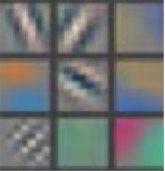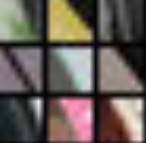# Layer2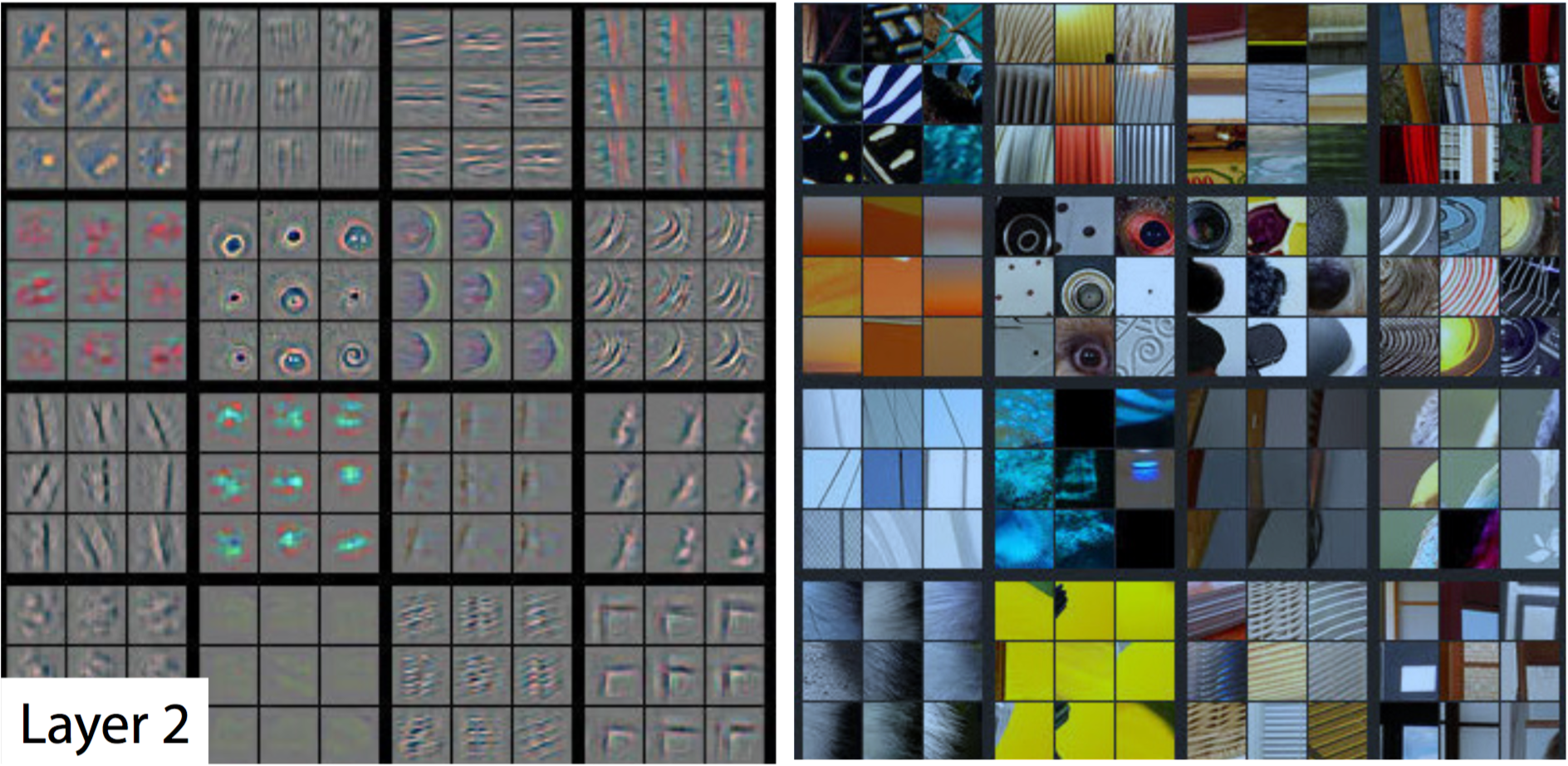# Layer3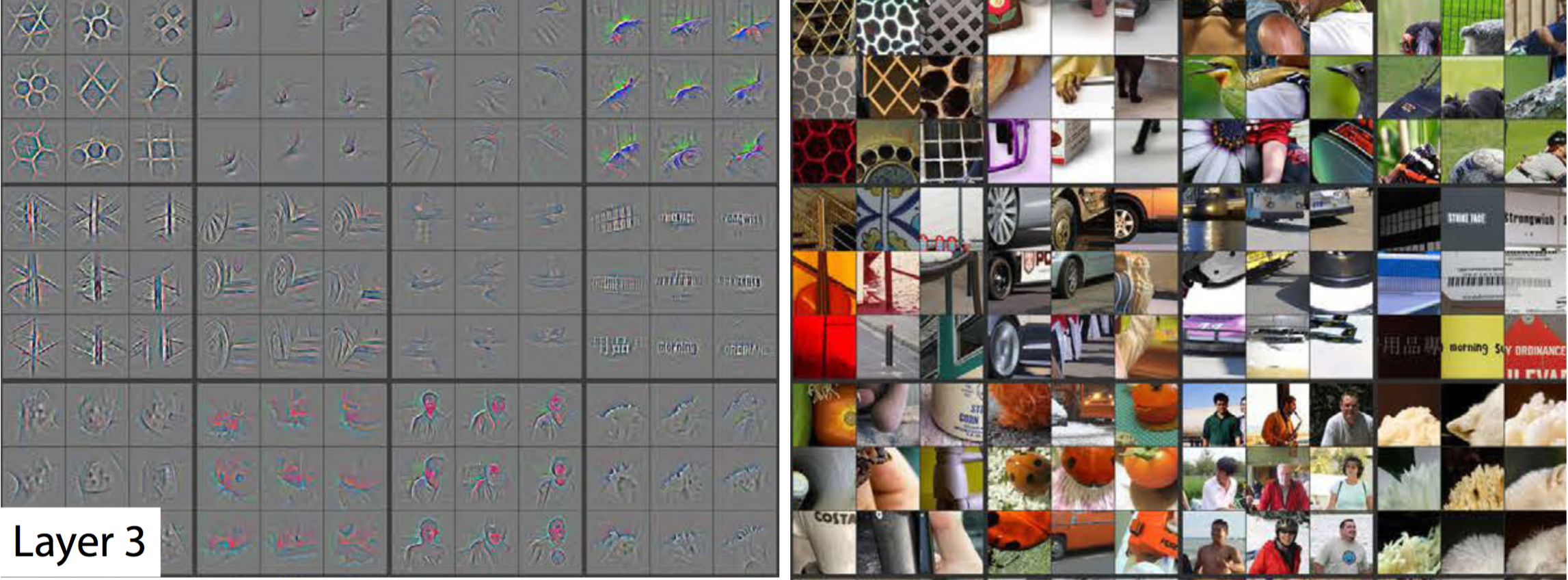# Layer5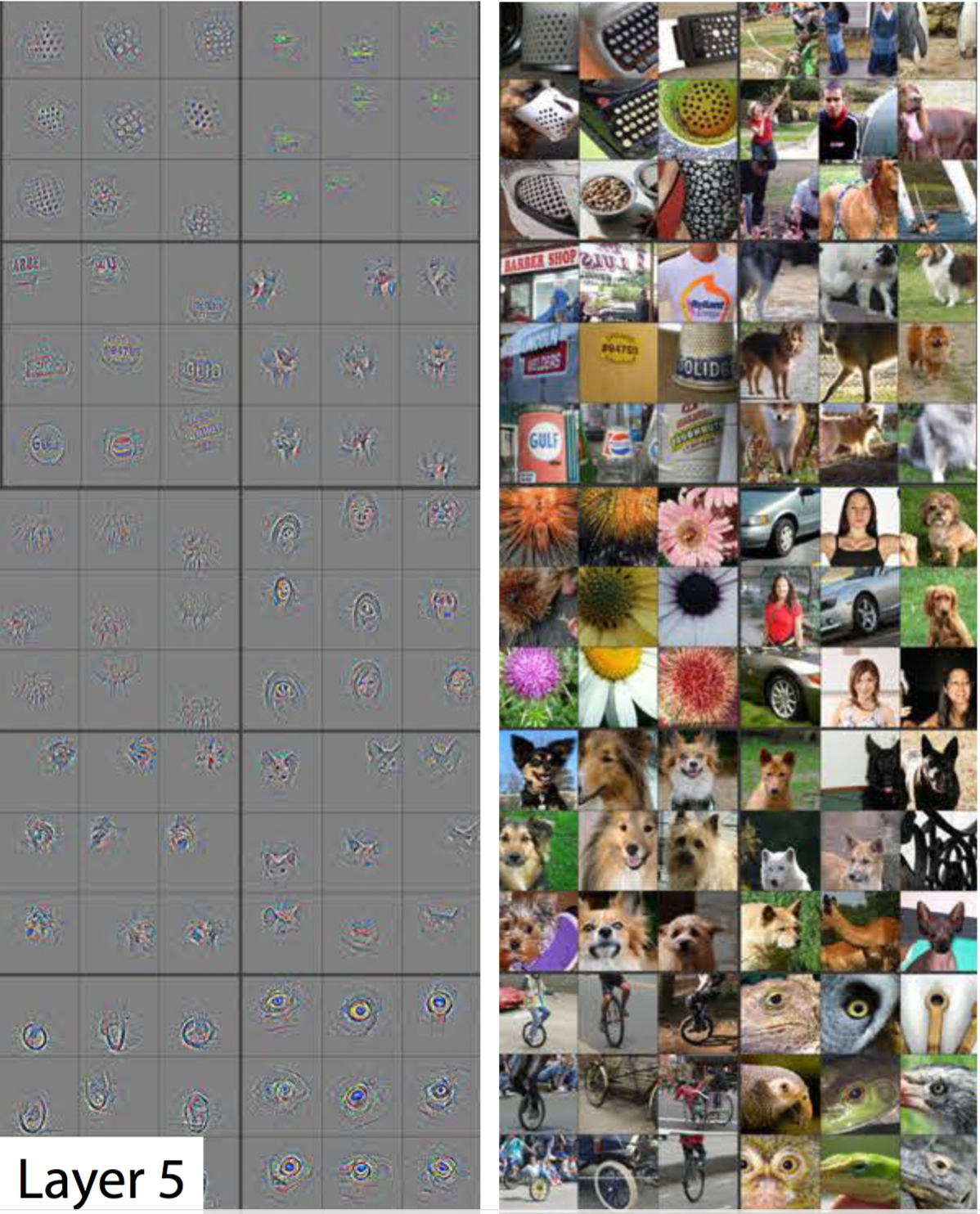# 在TensorFlow中使用CNN

``````# Output depth
k_output = 64

# Image Properties
image_width = 10
image_height = 10
color_channels = 3

# Convolution filter
filter_size_width = 5
filter_size_height = 5

# Input/Image
input = tf.placeholder(
tf.float32,
shape=[None, image_height, image_width, color_channels])

# Weight and bias
weight = tf.Variable(tf.truncated_normal(
[filter_size_height, filter_size_width, color_channels, k_output]))
bias = tf.Variable(tf.zeros(k_output))

# Apply Convolution
conv_layer = tf.nn.conv2d(input, weight, strides=[1, 2, 2, 1], padding='SAME')
# Apply activation function
conv_layer = tf.nn.relu(conv_layer)
``````

Github链接

# 更多文章请关注我的个人网站：

weiweizhao.com

## 05-01 CNN–直觉与滤波器

1. 把图像区分成很多个小的碎片
2. 识别某些固定的特征元素，例如眼睛，鼻子，毛发
3. 把这些元素都集合起来归类为某一种物品